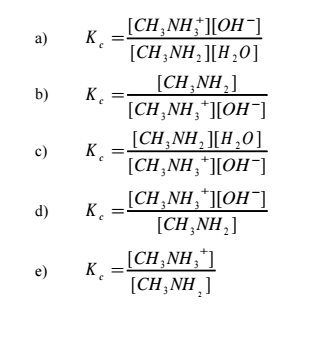# Problem: Methylamine is a weak base in water according to the equilibriumCH3NH2(aq) + H2O(l) ⇌ CH3NH3+(aq) + OH−(aq)What is the correct expression for the equilibrium constant?

###### FREE Expert Solution
86% (411 ratings)
###### Problem Details

Methylamine is a weak base in water according to the equilibrium

CH3NH2(aq) + H2O(l) ⇌ CH3NH3+(aq) + OH(aq)

What is the correct expression for the equilibrium constant?What scientific concept do you need to know in order to solve this problem?

Our tutors have indicated that to solve this problem you will need to apply the Equilibrium Expressions concept. If you need more Equilibrium Expressions practice, you can also practice Equilibrium Expressions practice problems.

What is the difficulty of this problem?

Our tutors rated the difficulty ofMethylamine is a weak base in water according to the equilib...as low difficulty.

How long does this problem take to solve?

Our expert Chemistry tutor, Jules took undefined 45 seconds to solve this problem. You can follow their steps in the video explanation above.

What professor is this problem relevant for?

Based on our data, we think this problem is relevant for Professor Dixon's class at UCF.